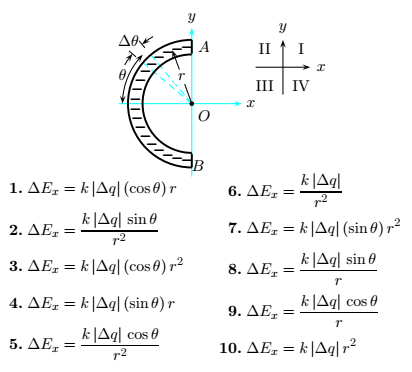# Problem: Consider the setup shown in the figure below, where the arc is a semicircle with radius r. The total charge Q is negative, and distributed uniformly on the semicircle. The charge on a small segment with angle ∆θ is labeled ∆q.  The magnitude of the x-component of the electric field at the center, due to ∆q, is given by

###### FREE Expert Solution
88% (245 ratings)
###### Problem Details

Consider the setup shown in the figure below, where the arc is a semicircle with radius r. The total charge Q is negative, and distributed uniformly on the semicircle. The charge on a small segment with angle ∆θ is labeled ∆q.  The magnitude of the x-component of the electric field at the center, due to ∆q, is given by